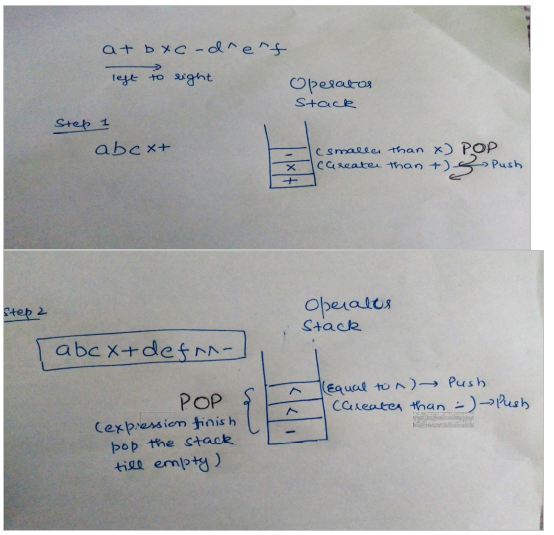Open in App
Not now

# GATE | GATE-CS-2004 | Question 90

• Difficulty Level : Easy
• Last Updated : 28 Jun, 2021

Assume that the operators +, -, × are left associative and ^ is right associative. The order of precedence (from highest to lowest) is ^, x , +, -. The postfix expression corresponding to the infix expression a + b × c – d ^ e ^ f is
(A) abc × + def ^ ^ –
(B) abc × + de ^ f ^ –
(C) ab + c × d – e ^ f ^
(D) – + a × bc ^ ^ def

Explanation: ^ is right assosciative.This solution is contributed by parul Sharma.

Quiz of this Question

My Personal Notes arrow_drop_up
Related Articles